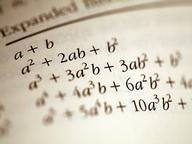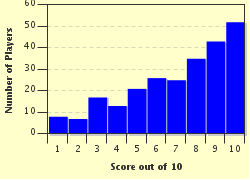FREE! Click here to Join FunTrivia. Thousands of games, quizzes, and lots more!# Fun with Quadratics! Trivia Quiz

### Who says that math can't be fun? Let's have some fun with everyone's favorite equations -- quadratics! :)

A multiple-choice quiz by Acj2011. Estimated time: 4 mins.

Author
Acj2011
Time
4 mins
Type
Multiple Choice
Quiz #
346,471
Updated
Dec 03 21
# Qns
10
Difficulty
Average
Avg Score
7 / 10
Plays
826
Last 3 plays: briarwoodrose (9/10), AdamM7 (10/10), catnlib (1/10).
This quiz has 2 formats: you can play it as a or as shown below.
Scroll down to the bottom for the answer key.
1. Using the standard quadratic form for 4x^2+7x-2, which of these is equal to -2? Hint

x
a
c
b

#### NEXT>

2. When writing the quadratic formula, you write that x equals -b plus or minus the square root of b^2-4ac, all divided by what? Hint

ab^2
2
c^2
2a

#### NEXT>

3. If you have the equation x^2-6x+9=0, how could you factor it? Hint

(x-6)(x+9)=0
(x-3)(x-3)=0
(x+6)(x-9)=0
(x-3)(x+3)=0

#### NEXT>

4. Multiply these two factors together to get a polynomial: (x-4)(x+3). Hint

x^2-12
x^2-x-12
-4x^2*3x+1
x^2+x-12

#### NEXT>

5. Use the quadratic formula to solve the following equation: x^2+4x-2=0. Hint

(-2+ square root 6, -2- square root 6)
(-2+ square root 2, -2+ square root 2)
(-4, 2)
(-2, 2)

#### NEXT>

6. Which of the following is a possible equation that would yield the solutions x=-2,5? Hint

x^2+2x-5=0
x^2+3x-10=0
x^2-3x-10=0
x^2-2x+5=0

#### NEXT>

7. When you have an equation, such as x^2-3x-4=0, which can be easily factored, it is not mathematically possible to use the quadratic formula to solve it.

True
False

#### NEXT>

8. Completing the square: When preparing to use this method, what must you first always ensure is true about your first coefficient (the coefficient of x^2)? Hint

There should be no x^2 term.
It should be negative
It should be equal to one
It should be greater than one

#### NEXT>

9. While completing the square, you must take the coefficient of the second term, divide it by two, then do what before adding it to the end of the polynomial? Hint

Square it
Use FOIL
Multiply it by the first term
Do nothing

#### NEXT>

10. Complete the square: x^2-10x+___=0. (Which of these numbers, inserted into the blank, makes the polynomial a perfect square?) Hint

-25
25
5
-5

 (Optional) Create a Free FunTrivia ID to save the points you are about to earn:Select a User ID:Choose a Password:Your Email:

Most Recent Scores
Sep 24 2023 : briarwoodrose: 9/10
Sep 23 2023 : AdamM7: 10/10
Sep 09 2023 : catnlib: 1/10

Score DistributionQuiz Answer Key and Fun Facts
1. Using the standard quadratic form for 4x^2+7x-2, which of these is equal to -2?

A quadratic in standard form is written ax^2+bx+c. Therefore, in this problem, the coefficients are a=4, b=7, and c=-2.
2. When writing the quadratic formula, you write that x equals -b plus or minus the square root of b^2-4ac, all divided by what?

The quadratic formula is written as: x equals -b plus or minus the square root of b^2-4ac all over 2a. This formula is derived by completing the square of the standard form of a quadratic equation, ax^2+bx+c=0.
3. If you have the equation x^2-6x+9=0, how could you factor it?

It is always easier to try to factor a problem before using the quadratic formula. After factoring this polynomial, you get (x-3)(x-3)=0, then you solve for x. The solution will be 3. If you use the quadratic formula, plugging in 1 for a, -6 for b, and 9 for c, you will ultimately come up with the same answer.
4. Multiply these two factors together to get a polynomial: (x-4)(x+3).

When multiplying two factors together you use the FOIL method. F stands for the First terms, O stands for the Outside terms, I stands for the inside terms, and L stands for the Last terms. This is the order in which you should multiply the terms of two factors. You then add these four products together to get the final, expanded expression.
5. Use the quadratic formula to solve the following equation: x^2+4x-2=0.

Answer: (-2+ square root 6, -2- square root 6)

For this problem, the coefficients are a=1, b=4, and c=-2. When you plug this into the quadratic formula, you get: -4 plus or minus the square root of 4^2-4*1*-2, all over 2*1. After solving this and simplifying the result, you are left with -2 plus or minus the square root of 6. Therefore, the answers are -2+ square root 6 and -2- square root 6.
6. Which of the following is a possible equation that would yield the solutions x={-2,5}?

In order to find the simplest equation that will yield these two solutions, use the reverse process used when solving a quadratic. Make the solutions into the two binomial factors, (x+2)(x-5). FOIL these two factors to multiply them together, and you will get x^2-3x-10. So, your equation is x^2-3x-10=0.
7. When you have an equation, such as x^2-3x-4=0, which can be easily factored, it is not mathematically possible to use the quadratic formula to solve it.

While it is much easier to simply factor the polynomial into (x-4)(x+1)=0, it is still possible to use the quadratic formula. Most people choose not to use the quadratic formula in such cases, however, because it is more tedious to plug the numbers into the formula. However, if you truly enjoy math, by all means, you can use the quadratic formula every single time!
8. Completing the square: When preparing to use this method, what must you first always ensure is true about your first coefficient (the coefficient of x^2)?

Answer: It should be equal to one

When preparing to complete the square, you must firstly make sure that your equation is in standard quadratic form with the right-hand side set equal to zero. Then, always make sure that the coefficient of the first term is positive one. Otherwise, your calculations will be incorrect.
9. While completing the square, you must take the coefficient of the second term, divide it by two, then do what before adding it to the end of the polynomial?

You must divide the coefficient of the second term in half and then square it. After that, you can add it to the end of your polynomial. After completing the square, you should have an equation with two of the same factors. For instance, for the polynomial x^2+2x+1, the two factors are (x+1)(x+1), or (x+1)^2. Therefore it is a perfect-square trinomial.
10. Complete the square: x^2-10x+___=0. (Which of these numbers, inserted into the blank, makes the polynomial a perfect square?)

The quadratic equation is in standard form and the first coefficient is positive and is equal to one, so we are ready to begin the problem. Take the coefficient of the second term, 10. Divide it in half (10/2=5), then square the result (5^2=25). Your answer is 25.
Source: Author Acj2011

This quiz was reviewed by FunTrivia editor CellarDoor before going online.
Any errors found in FunTrivia content are routinely corrected through our feedback system.
Related Quizzes
1. The Matrix Average
2. An Adventure in Abstract Algebra Very Difficult
3. Basic Math or Algebra? Tough
4. Algebra Wonderland! Tough